Examples from NCERT Book

Class 10
Chapter 12 Class 10 - Electricity

## 100 J of heat is produced each second in a 4 Ω resistance. Find the potential difference across the resistor.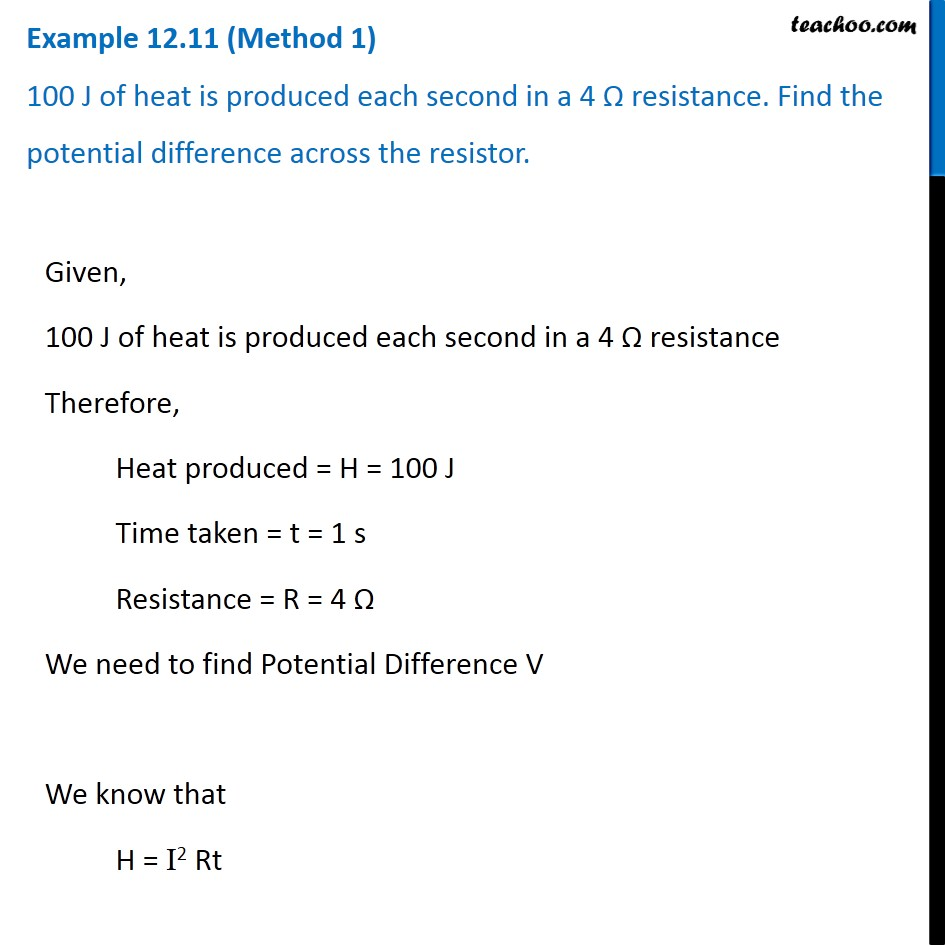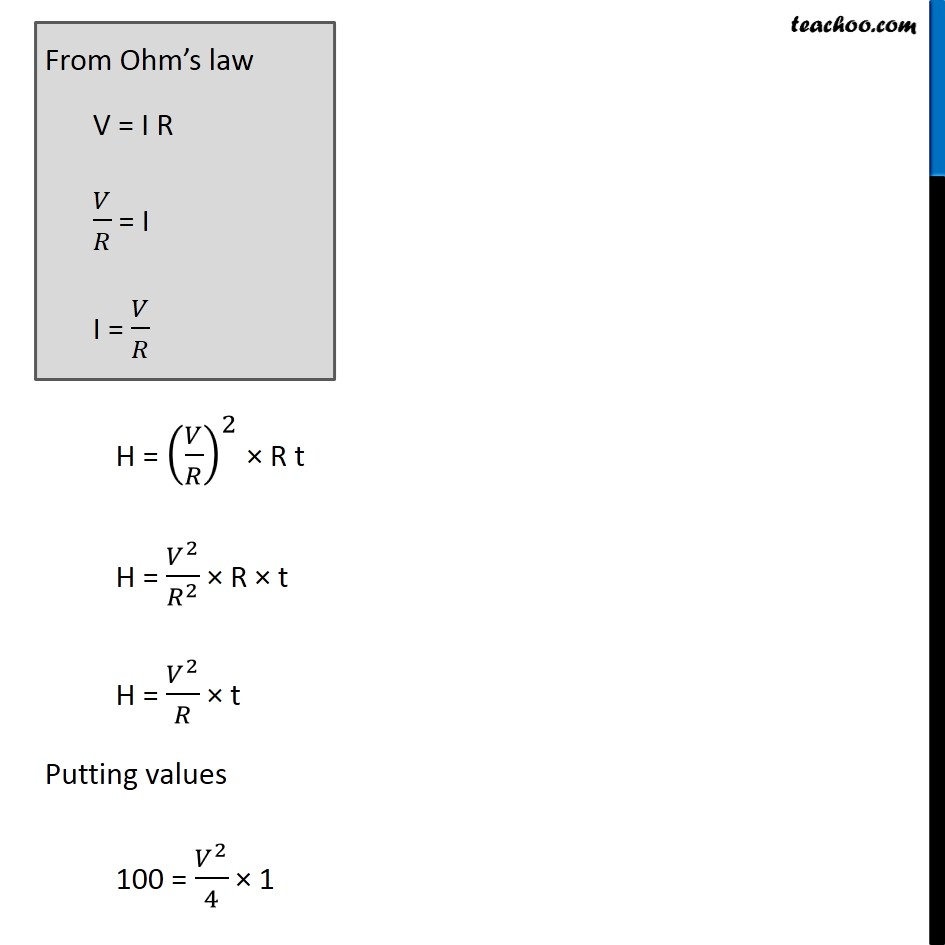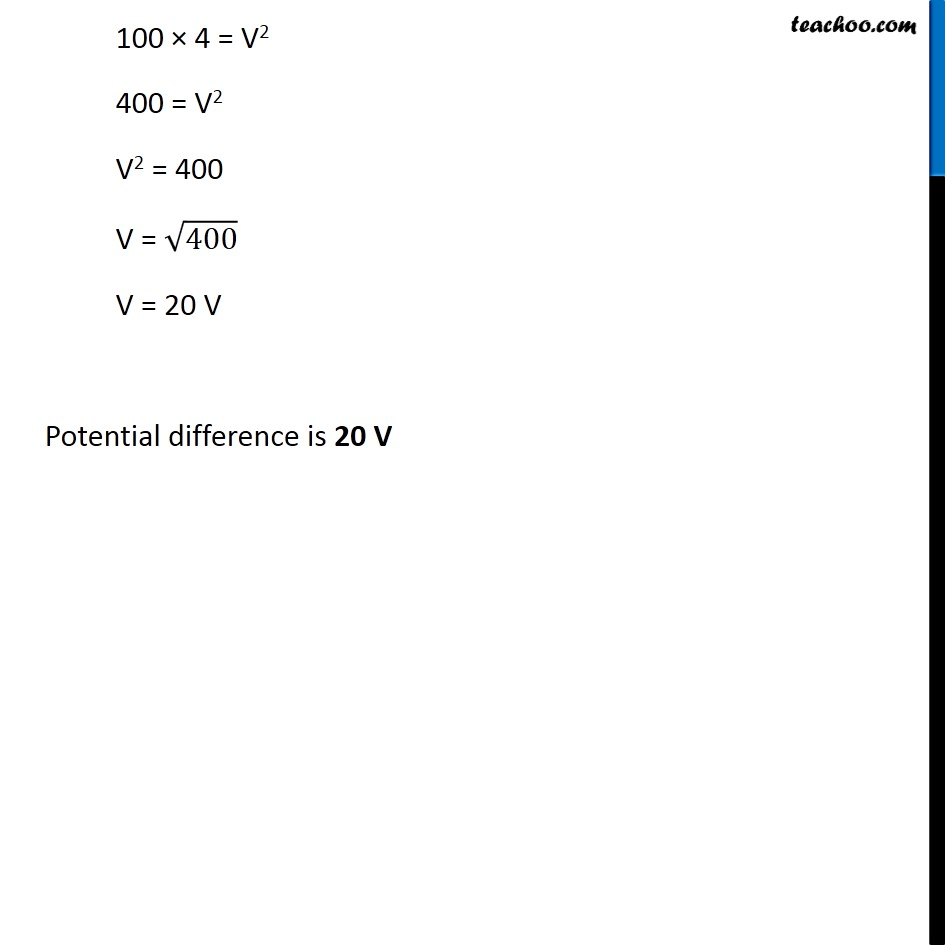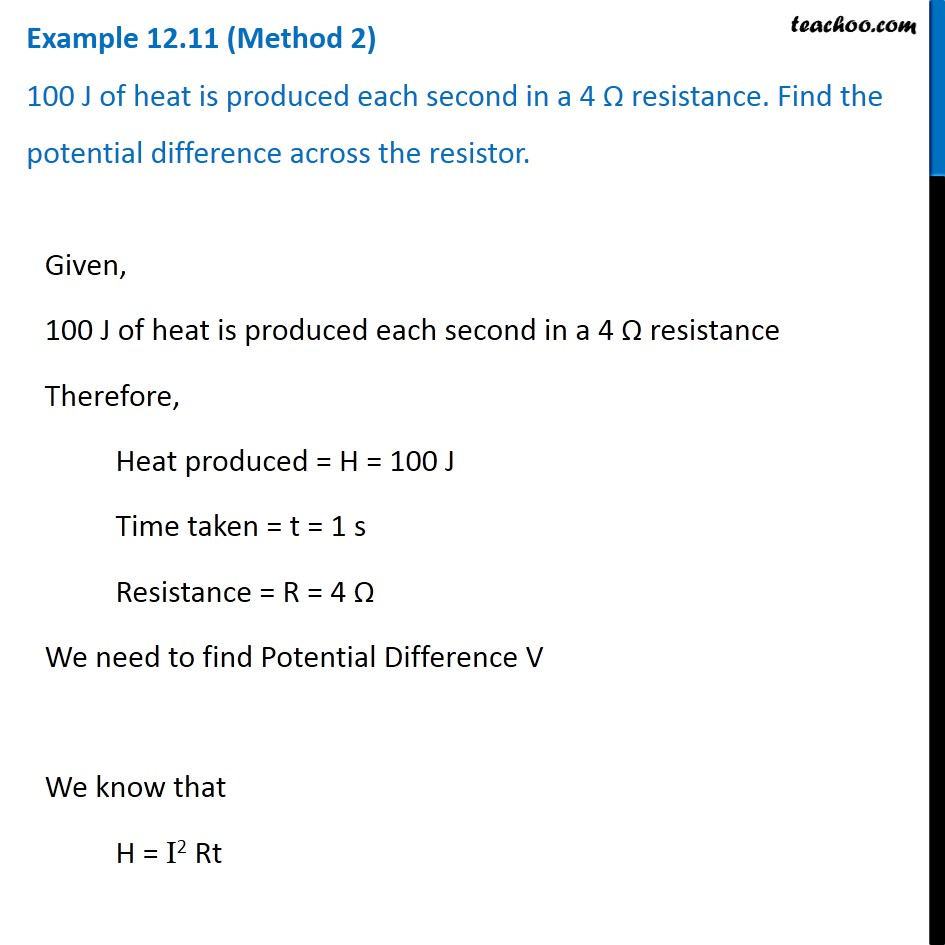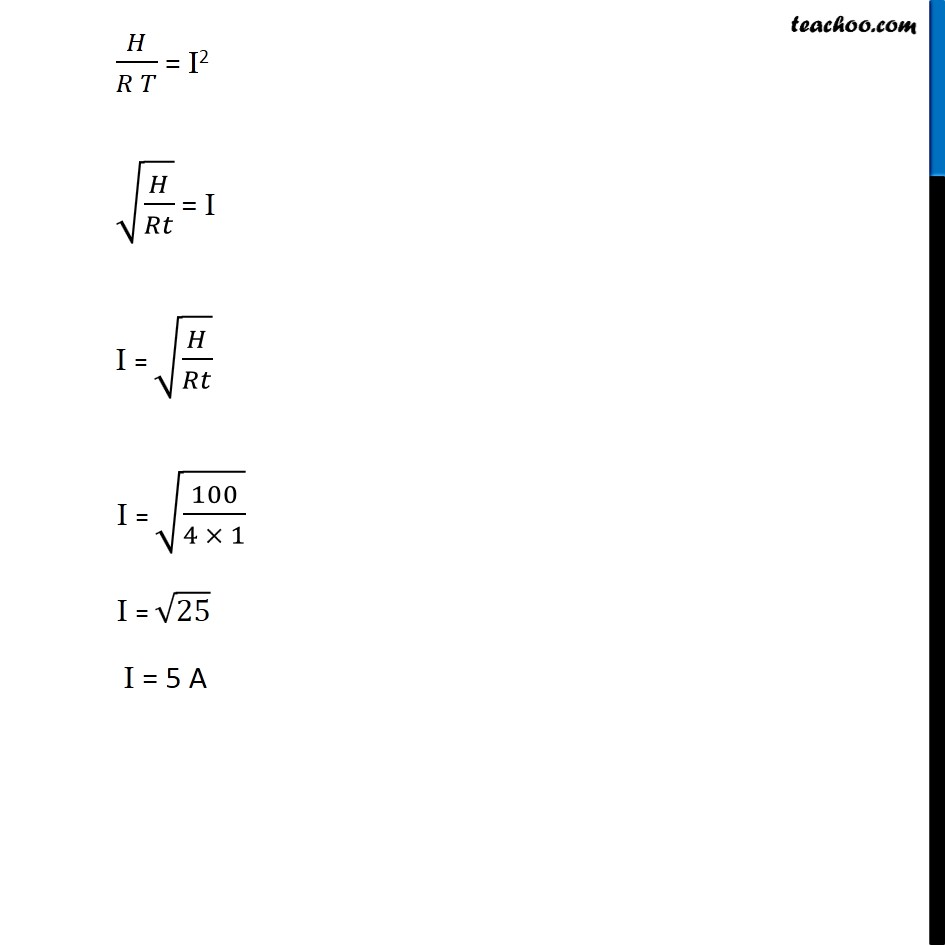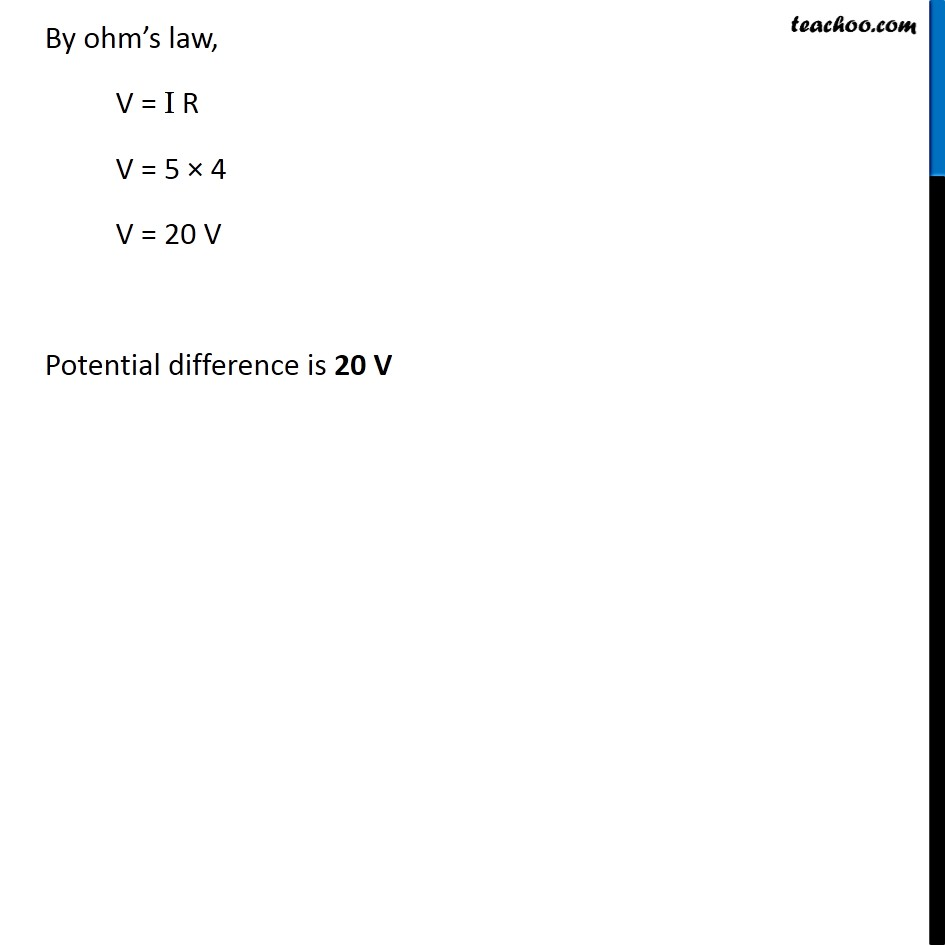Learn in your speed, with individual attention - Teachoo Maths 1-on-1 Class

### Transcript

Example 12.11 (Method 1) 100 J of heat is produced each second in a 4 Ω resistance. Find the potential difference across the resistor. Given, 100 J of heat is produced each second in a 4 Ω resistance Therefore, Heat produced = H = 100 J Time taken = t = 1 s Resistance = R = 4 Ω We need to find Potential Difference V We know that H = I2 Rt From Ohm’s law V = I R 𝑉/𝑅 = I I = 𝑉/𝑅 H = (𝑉/𝑅)^2 × R t H = 𝑉^2/𝑅^2 × R × t H = 𝑉^2/𝑅 × t Putting values 100 = 𝑉^2/4 × 1 100 × 4 = V2 400 = V2 V2 = 400 V = √400 V = 20 V Potential difference is 20 V Example 12.11 (Method 2) 100 J of heat is produced each second in a 4 Ω resistance. Find the potential difference across the resistor. Given, 100 J of heat is produced each second in a 4 Ω resistance Therefore, Heat produced = H = 100 J Time taken = t = 1 s Resistance = R = 4 Ω We need to find Potential Difference V We know that H = I2 Rt 𝐻/(𝑅 𝑇) = I2 √(𝐻/𝑅𝑡) = I I = √(𝐻/𝑅𝑡) I = √(100/(4 × 1)) I = √25 I = 5 A By ohm’s law, V = I R V = 5 × 4 V = 20 V Potential difference is 20 V